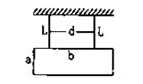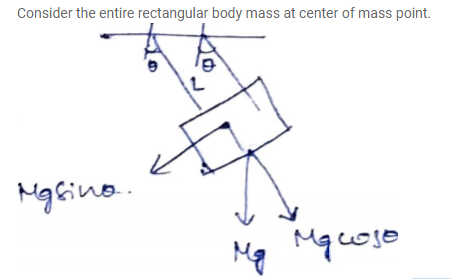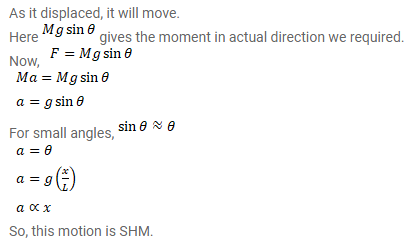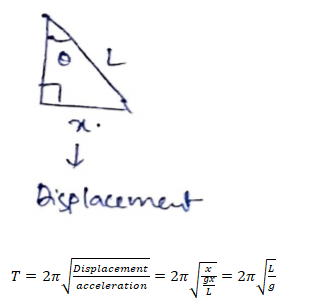# A rectangular plate of sides a and b is suspended from

Question:

A rectangular plate of sides $a$ and $b$ is suspended from a ceiling by two parallel strings of length $L$ each. The separation between the strings is $d$. The plate is displaced slightly in its plane keeping the strings tight. Show that it will execute simple harmonic motion. Find the time period.Solution: